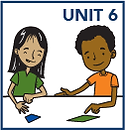## Grade 3

In grade 3 math, your child will:

• use addition, subtraction, multiplication, and division to solve story problems

• solve multiplication and division facts accurately and efficiently

• multiply 1-digit numbers by multiples of 10, e.g., 3 x 70

• add and subtract 2- and 3-digit numbers

• compare, recognize, and generate equivalent fractions and place them on a number line

• estimate and measure in time, liquid volume, and masses of objects

• round numbers to nearest 10 or 100

• tell time to the minute

• estimate and measure liquid volume and mass in metric units

• solve area and perimeter problems

• identify and construct different kinds of quadrilaterals

• sort and classify shapes